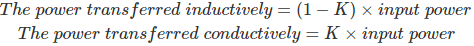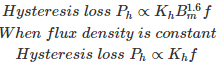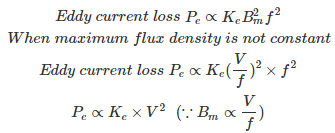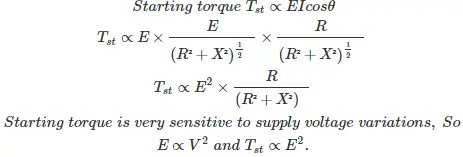# MCQs on Electrical Machines

##### Page 44 of 114. Go to page 1 2 3 4 5 6 7 8 9 10 11 12 13 14 15 16 17 18 19 20 21 22 23 24 25 26 27 28 29 30 31 32 33 34 35 36 37 38 39 40 41 42 43 44 45 46 47 48 49 50 51 52 53 54 55 56 57 58 59 60 61 62 63 64 65 66 67 68 69 70 71 72 73 74 75 76 77 78 79 80 81 82 83 84 85 86 87 88 89 90 91 92 93 94 95 96 97 98 99 100 101 102 103 104 105 106 107 108 109 110 111 112 113 114
01․ A 10KVA auto transformer, turn ratio is 0.4. Find the power transferred inductively?
4 KVA
6 KVA
10 KVA
0 KVA

Power transferred inductively = (1-K) × input power = 0.6×10×103 = 6 KVA

02․ A 20KVA auto transformer, turn ratio is 0.8. Find the power transferred conductively?
16KVA
4KVA
20KVA
0KVA

Power transferred conductively = K × input power = 0.8× 20 KVA = 16 KVA

03․ In an auto transformer, for what value of turn ratio, power transferred by inductively and conductively are same?
1
0
0.5
any of the aboveTherefore, when turn ratio is 0.5 (K=1-K), the power transferred inductively and conductively are same.

04․ The total core loss of a 400 V, 50 Hz transformer is 2400W, when it is supplied by 200 V, 25 Hz loss is 800W. Calculate eddy current loss and hysteresis loss?
1000W , 1400W
1600W, 800W
800W, 1600W
1400W, 1000W

Eddy current loss Pe ∝ KeBm²f² Hysteresis loss Ph ∝ KhBm1.6f For given problem Bm is constant. When Bm is constant, Hysteresis loss is proportional to frequency and eddy current loss is proportional to square of the frequency. Power loss P = Pe+Ph Bm= 400/50 = 200/25 = 8 From given data, 2400 = Ke×50²+ Kh×50 800 = Ke×25²+Kh×25 From the above equations, Ke = 0.64, Kh = 16 Eddy current loss Pe = 0.64×50² = 1600W Hysteresis loss Ph = 16×50 = 800 W

05․ In a transformer which of the following losses are zero?
Iron loss
Copper loss
Rotational loss
All of the above

Transformer is a static device and there are no moving parts. Therefore rotational losses are zero in transformer.

06․ When maximum flux density is constant, the eddy current losses are proportional to
f
f2
V
V2

Eddy current loss Pe ∝ Ke Bm2f2 When maximum flux density is constant, Eddy current loss, Pe ∝ f2

07․ When flux density is constant in a transformer, hysteresis loss varies as
f
V08․ In a transformer maximum flux density is not constant, the eddy current loss varies as
f
V09․ To increase starting torque of a 3-phase slip ring induction motor, preferred method is to increase
supply voltage
supply frequency
rotor resistance
none of the aboveInduction motor is termed as rotating transformer with short circuited secondary. Rated voltage can not be applied across the stator because as the rotor is stationary high voltage induced in the rotor. It is similar to applying rated voltage across the transformer primary when secondary is short circuited. The motor draws 7 to 10 times the rated current during starting. Therefore, induction motor can not start with rated voltage to get high starting torque. Starting torque is also proportional to rotor resistance (Tst∝R.), by increasing of rotor resistance starting torque will improve. This technique is used in slip ring induction motors to get high starting torque.

10․ In single phase rotary converter the number of slip rings will be
two.
three.
four.
six.

The single phase power supply has two terminals that are phase and neutral. So, two slip rings are enough to provide the power supply to the single phase rotary converter.

<<<4243444546>>>